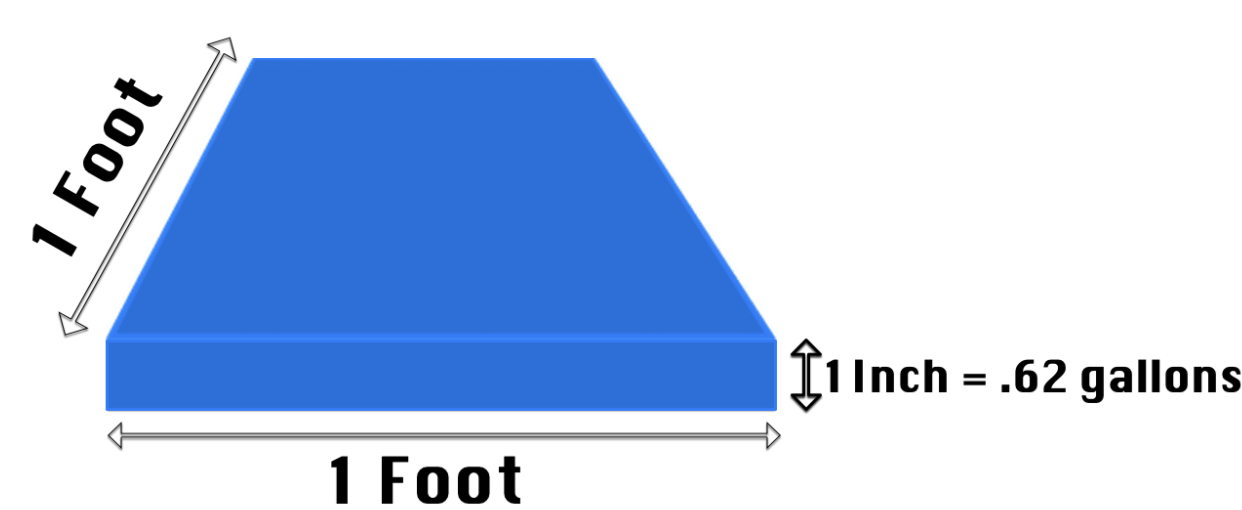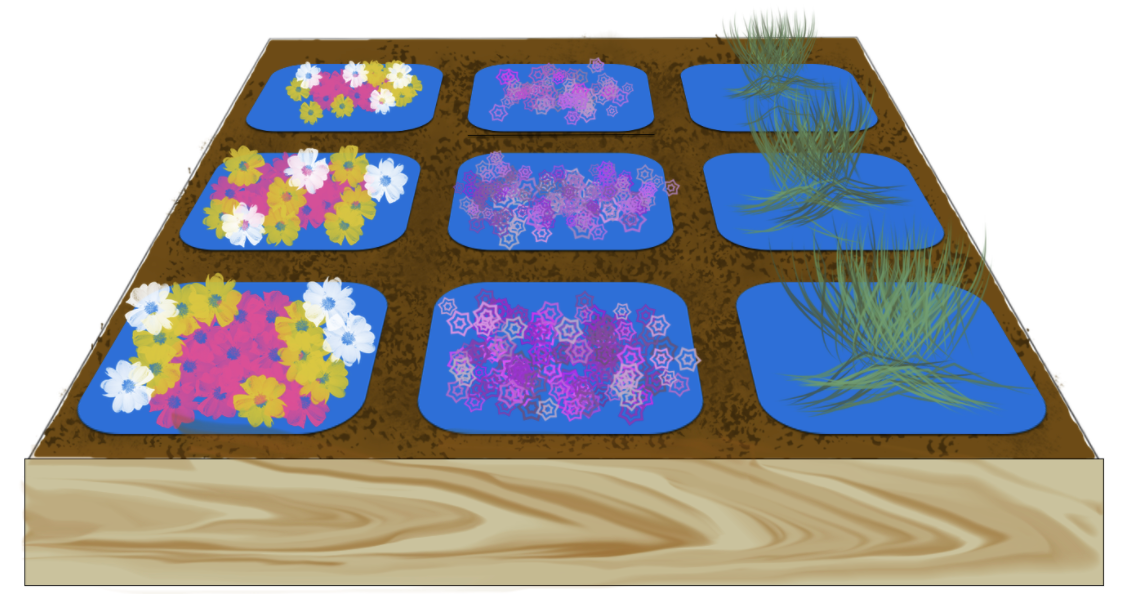How do I convert inches to gallons?

To determine how many gallons of water you need to provide a specific amount of inches of water to your system, you will need to first determine how many square feet are in the area you want to water.

To begin, .62 gallons of water make up 1 inch in a square foot.Imagine you have a 3’ x 3’ raised bed; with 9 square feet total that needs to be watered. When calculating inches to gallons, it is best to think of each of these square feet as empty tiles. Let’s say you want to provide 2” of water to the raised bed:First, multiply the .62 gallons of water for every inch needed (.62 gallons x 2 inches) = 1.24 gallons for 2” of water per square foot.

Next, multiply the gallons of water (1.24) by the number of square feet in the bed (9 square feet); 1.24 gallons per foot x 9 square feet = 11.16 gallons of water for 2 “ in a 9 square foot bed.

We now know 11.16 gallons of water is needed to supply 2” of water to the 3’ x 3’ bed.

How long do I need to water for?

This will depend on the flow rate of your watering devices. Let’s say in the 3’ x 3’ raised bed you are using ¼” dripline with 6” emitter spacing, .5 GPH drippers, and there are a total of 3 runs per bed.

If each run is 3 feet long (3 feet x 12 inches = 36”) and has 6” emitter spacing (36” / 6”) would mean there are 6 emitters per 3 foot run. If each emitter is .5 GPH (6 emitters x .5 GPH) that would be 3 GPH per line.

If you have 3 GPH per line, and there are a total of 3 lines (3 GPH x 3 lines) that comes out to 9 GPH total.

We know we need 11.16 gallons total to provide the 2” needed. 11.16 gallons / 9 gallons per hour = 1.24 hours of watering will be needed.

How much time is 1.24 hours?

(1.24 hours x 60 minutes = 74.4 minutes, or 1 hour and 15 minutes)

What if I don’t want to provide all 2” or 11.16 gallons of water at once? What if I want to supply that over a week?

If you want to water multiple times a week simply break that 74.4 minutes up as needed. Let’s say you want to water 3 times per week. 74.4 minutes / 3 watering times = 24.8 minutes per watering duration (if watering 3 times a week).

Still, have questions? Our customer service agents are happy to help. Please reach out to our